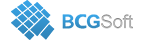BCGControlBar Pro for MFC
 void CBCGPChartSeries::SetVirtualMode ( BOOL bSet, BCGPCHART_VSERIES_CALLBACK pCallBack, LPARAM lParam = `NULL`, double dblMinRange = `0.`, double dblMaxRange = `0.`, BOOL bRedraw = `TRUE` )

Enables virtual mode for a series.

Call this method to enable "virtual" mode for a series. In this mode a series does not contain data points. Instead, screen coordinates of the series curve are calculated by a custom pCallback callback method, which is called for each X value that can be currently displayed on the X axis.

The Chart itself takes care of sending X values with a step corresponding to each pixel on the X axis. As a result the series will be drawn as a non-discrete curve according to the formula implemented in the callback:

Note. If dblMinRange is equal to dblMaxRange, the series assumes that the range is not set and uses the current minimum and maximum calculated on the X axis from minimums and maximums of other series. If no other series displayed, you must set the range or call CBCGPChartAxis::SetFixedDisplayRange.

The callback has the following prototype:

typedef CBCGPChartValue (CALLBACK* BCGPCHART_VSERIES_CALLBACK)(double dblXValue, CBCGPChartSeries* pVirtualSeries, LPARAM lp);

If a formula can't calculate a value for the specified dblXValue (for example, log(x) can be calculated only for x > 0), the callback must return an empty value.

Parameters
 bSet TRUE - turn the virtual mode on; FALSE - turn the virtual mode off pCallBack A pointer to a custom callback that implements a formula y = f(x). lParam User-defined data to pass to the callback. dblMinRange Specifies minimum range value. dblMaxRange Specifies maximum range value. bRedraw Redraws the chart.

The following example illustrates a series in virtual mode that displays on the diagram a formula y = sqrt(x) in range x = [0, 100]:

Copy
CBCGPChartSeries* pSeries = pChart->CreateSeries(_T("Virtual Series"));
pSeries->SetVirtualMode(TRUE, ChartCallbackFunc, NULL, 0, 100);
CBCGPChartValue CALLBACK ChartCallbackFunc(double dblXValue, CBCGPChartSeries* pFormulaSeries, LPARAM lp)
{
CBCGPChartValue valResult;
if (dblXValue > 0)
{
valResult = sqrt(dblXValue);
}
return valResult;
}